# Memorize Frequently Used Expenses

If your organization has activated this feature, you may "memorize" frequently used expense line items in order to easily add them to future reports from the eWallet. For example, if you create the same Internet Charges expense every month, you would be able to memorize that line item to have it created as an Offline transaction in the eWallet for future use.

• Note that itemized expenses like Hotels may not be memorized.

## Create Memorized Expense

1. After you have saved the line item you wish to memorize, click the MORE (three dots) button on the Line Item preview and select MEMORIZE EXPENSE.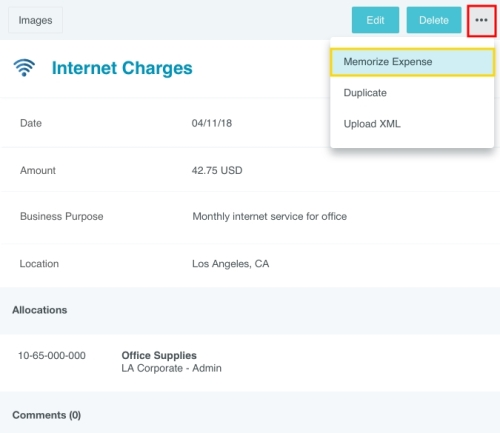2. You will be asked to name the memorized expense so you can easily find it in the future. Then click CREATE.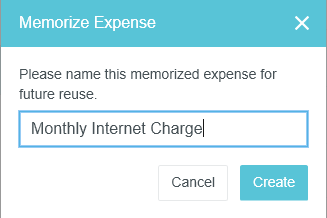3. The expense will now be saved in the Offline section of the eWallet for future use.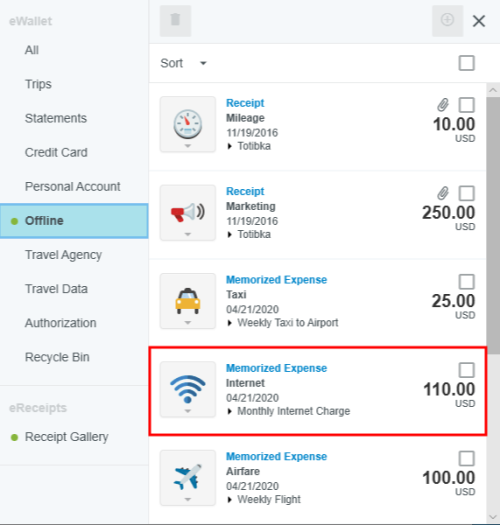## Add Memorized Expenses to Report

1. Click the plus button to add a line item, then click OFFLINE in the eWallet.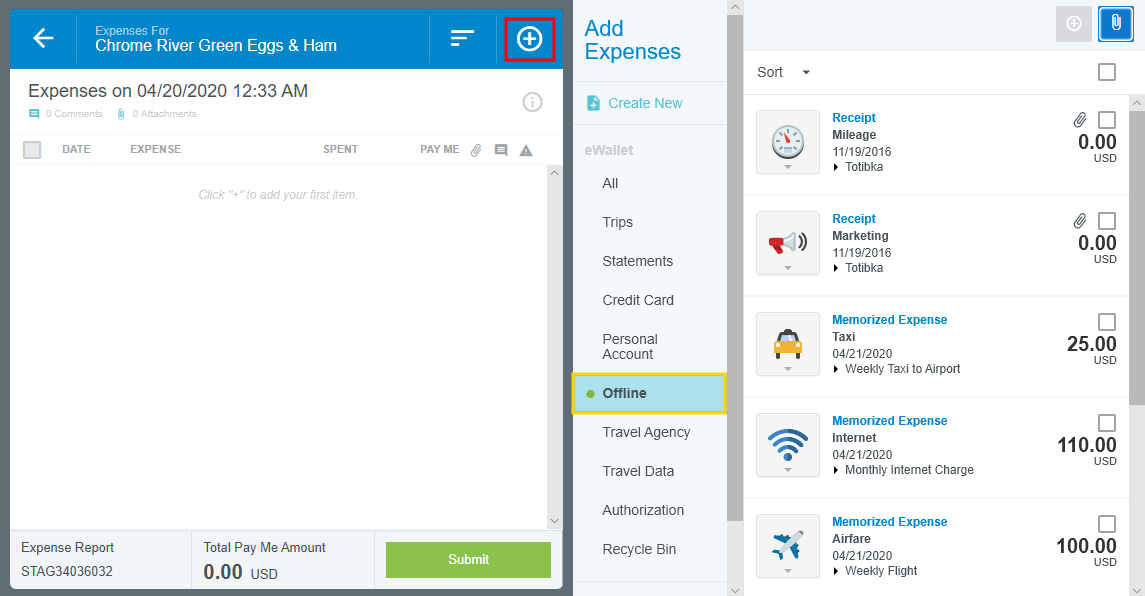2. Find the desired memorized expenses and click the check boxes to select them. Then click the ADD or PLUS button.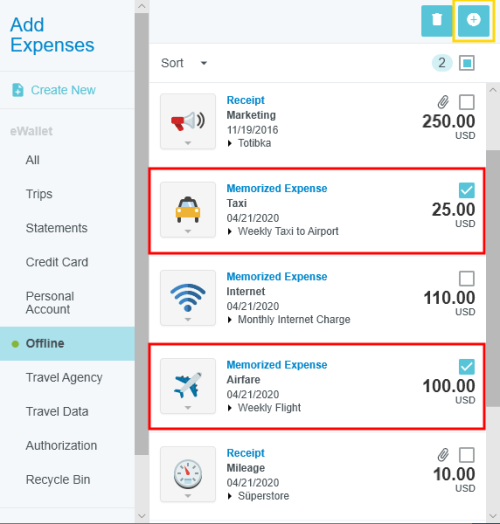3. Each memorized expense will open in edit mode to allow you to make any necessary adjustments and save the line item to the new report.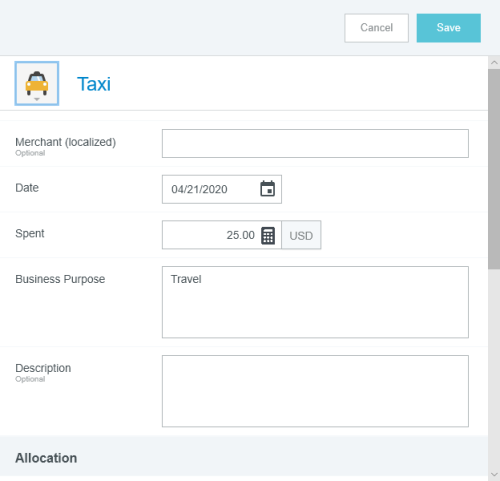4. The original memorized expenses will remain in the eWallet for future use.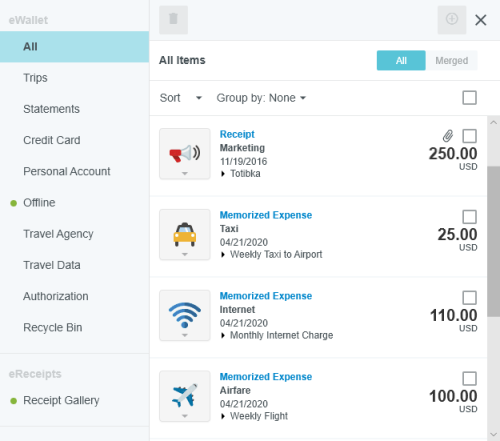<% if (allItems.length > 1) { %>

<% } %>
<% var getColumnClasses = function(numberColumns) { var classNames = 'col-12'; if (numberColumns >= 2) classNames += ' md:col-6'; if (numberColumns >= 3) classNames += ' lg:col-4'; if (numberColumns >= 4) classNames += ' xl:col-3'; return classNames; } %>

##<%= heading %>

<% } %>
<% var getColumnClasses = function(numberColumns) { var classNames = 'col-12'; if (numberColumns >= 2) classNames += ' md:col-6'; if (numberColumns >= 3) classNames += ' lg:col-4'; if (numberColumns >= 4) classNames += ' xl:col-3'; return classNames; } %>

##<%= heading %>

<% } %>
<% var maxSections = 5 %>
<% if (sections.length) { %>
<% sections.forEach(function(section) { %>
• <%= partial('partial-article-list-sections', { id: 'section-' + section.id, parentId: '#' + id, sections: section.sections, activeCategoryId: activeCategoryId, activeSectionId: activeSectionId, activeArticleId: activeArticleId, partial: partial }) %> <% if (section.articles.length) { %> <% } %>
• <% }); %>
<% } %>
<% if (sections.length) { %>
<% sections.slice(0, maxSections).forEach(function(section) { %>
• <%= section.name %> <%= partial('partial-section-list-sections', { parent: section, sections: section.sections, maxSections: maxSections, partial: partial }) %>
• <% }); %> <% if (sections.length > maxSections) { %>
• See more
• <% } %>
<% } %>
<% var getColumnClasses = function(numberColumns) { var classNames = numberColumns === 'auto' ? 'col-auto' : 'col-12'; if (numberColumns >= 2) classNames += ' md:col-6'; if (numberColumns >= 3) classNames += ' lg:col-4'; if (numberColumns >= 4) classNames += ' xl:col-3'; return classNames; } %>
<% blocks.forEach(function(block, index) { %>
• <% if (imageHeight) { %><% } %>
<% if (block.name) { %>

<% if (block.html_url) { %> <%= block.name %> <% } else { %> <%= block.name %> <% } %>

<% } %> <% if (block.description) { %>

<%= block.description %>

<% } %>
• <% }) %>